M328K First Midterm Exam, February 21, 2003

1. Using induction, prove the formula: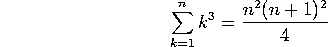2. As you know, the Fibonacci numbers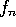are defined by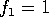,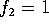and, for n>2,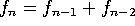. Give a rigorous proof of the assertion: ``is divisible by 3 if and only if n is divisible by 4.'' [Hint: Before writing down your proof, you may want to first determine which Fibonacci numbers are congruent to 1 (mod 3), which are congruent to 2 (mod 3), and which are divisible by 3. I'm sure you'll see the patters quickly enough.]

3. Greatest common factors:

a) Find the greatest common factor of 66 and 52.

b) Write this number explicitly as a linear combination of 66 and 52. For instance, if (66,52) were equal to 24 (which it obviously isn't!), you might write ``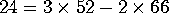''.

c) What is the least common multiple of 66 and 52?

4. Congruences, Diophantine equations and the Chinese Remainder Theorem.

a) Find all integer solutions to the equation 25 x + 38 y = 1.

b) Find a solution to the equation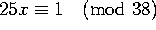.

c) Find a solution to the equation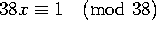.
(This is a typo. The question should have been (mod 25), not (mod 38))

d) Find a positive solution to the congruences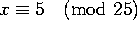,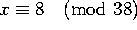.